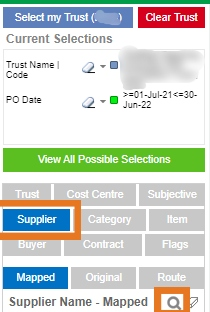Tail-end spend can account for a high amount of spend within organisations. It is possible to view this spend within Procurement Dashboard with the use of a search formula. For the example below we are assuming tail-end spend to be defined as spend between £0-£5000, these numbers can easily be changed within the formulas.

Depending on how you would like to evaluate your spend there are 3 formulas that can help:

• Where invoice spend is between £0 and £5000
`=sum([Invoice Line Net Amount])>0 and sum([Invoice Line Net Amount])<5000`

• Where invoice and PO combined spend is between £0 and £5000
`=(sum([PO Line Net Amount])+sum([Invoice Line Net Amount]))>0 and (sum([PO Line Net Amount])+sum([Invoice Line Net Amount]))<5000`

• Where PO spend is between £0 and £5000
`=sum([PO Line Net Amount])>0 and sum([PO Line Net Amount])<5000`

1) Add your desired filters, for example, a date range that you wish to analyse

2) Whether you wish to look at Tail-end spend in terms of spend with supplier or for products, you need to select the relevant filter. The example below is looking into suppliers with PO spend between £0 - £5000 between 1st July 2021 and 30th June 2022.3) Within the relevant filter pane select the magnifying glass, remove the wild cards ("**") and paste the required formulas from above, press enter and wait for the filters to be applied.4) This new filter will then appear within your filter pane as below.